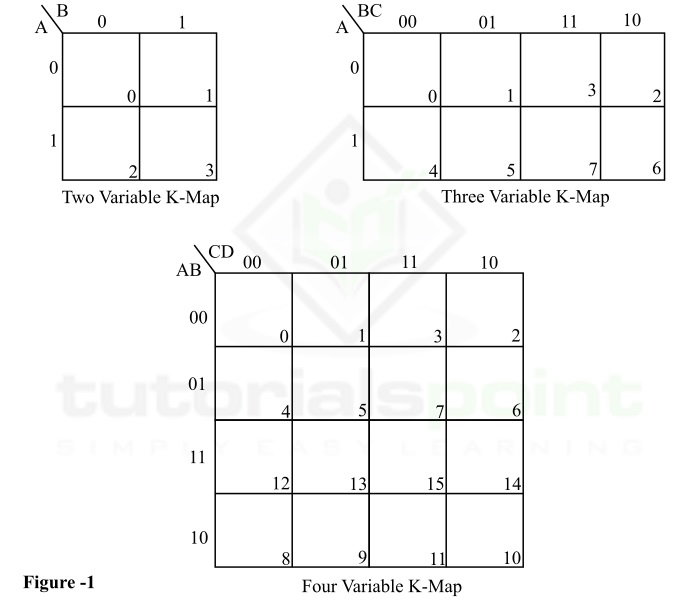# Karnaugh Map and its Limitations

## What is Karnaugh Map?

In realization of digital electronic systems, the simplification of Boolean expressions is one of the most crucial steps because it reduces the hardware complexity and cost of production. There are several tools and methods available for simplifying complex Boolean expression. K-Map or Karnaugh Map is one of such simplification methods. K-Map was developed by Maurice Karnaugh in the year of 1953. It is a visual or graphical method used to simplify the Boolean expressions.

K-Map is one of the most efficient simplification tools when the number of variables in the Boolean expression are less than or equal to four. However, for five, six, and more variables, the K-Map becomes quite difficult.

The K-Map or Karnaugh map makes the use of two dimensional table for simplification of the Boolean functions. The size of this table increases considerably with the increase in the number of variables in the Boolean functions.

Some typical examples of K-Map of two variable, three variable, and four variables are shown in Figure-1.From Figure-1, it is clear that the number of squares or cells in the Karnaugh map depends on the number of variables in the expression.

If n is the number of variables in the given Boolean function, then the corresponding Karnaugh map (K-Map) will have 2n squares or cells. For examples, if the number of variables in the Boolean function is 3, then the corresponding K-Map will have 8 (= 23) cells.

## Structure of Karnaugh Map

All the Karnaugh maps or K-Maps have a generalized similar structure as shown in Figure-1. A typical K-Map has a table of certain cells. On the top-left corner of this table, a set of variables are represented as A, B, C, D. These variables are basically the input variables involved in the logical expression that requires to be simplified.

The values of these inputs variables in binary form are represented along their respective sides, i.e., on the top and left of the table.

From the above examples, it can be observed that the binary numbers along the top and left of the K-Map are not in their normal binary order, instead they are in the gray code. The Gray code is used to ensure that the two physically adjacent cells are actually adjacent. This makes the process of grouping easier during minimization of the Boolean expression.

For providing simplicity in reading the K-Map, each cell of the K-Map is assigned a decimal number represented at the bottom-right corner of the cell. For example, in the three variable K-map (figure-1), the second cell of the K-Map represents a bit pattern 001, hence this cell is represented by its decimal equivalent 1.

## K-Map Simplification

The procedure of K-Map or Karnaugh map simplification is started with the entering the values of the variables, either in their SOP (Sum of Products) form or in POS (Product of Sums) form, in the right K-map cells. After that we need to group the maximum number of 1s (in the case of SOP form) or the maximum number of 0s (in the case of POS form). Each of these groups must be in powers of 2 and must be carried on in decreasing order only.

Once the grouping is done, each group has to be expressed in terms of combinations of input variables which are corresponding to the common binary values along the associated rows and columns. At last, all the combinations express the output expression of the Boolean function.

The following are the important advantages of the Karnaugh map −

• For simplifying Boolean expression, the K-map does not require the knowledge of theorems of Boolean algebra.

• Karnaugh map involves less number of steps in simplification process of logical expressions as compared to other simplification techniques.

## Limitations of Karnaugh Map

The following are the major limitations of the Karnaugh map −

• The most significant limitation of the Karnaugh map is that it is only efficient when the Boolean expression has less number of variables. It becomes quite complicated with the higher number of variables in the logical expression.

• The simplification of a Boolean function having more than or equal to five variables using K-Map is quite complex.

• It is very difficult to get equations correct with more than 5 variables using the K-map.

## Conclusion

The Karnaugh map or K-Map is an efficient tool for simplifying Boolean expression up to 4 variables. It is an easy method for simplification of logic expression because it does not make the use of Boolean algebra theorems. Another advantage of K-Map is that it is a visual method of simplification. However, the K-map becomes complex and inefficient when the variables in the logical expression are equal to or more than 5.